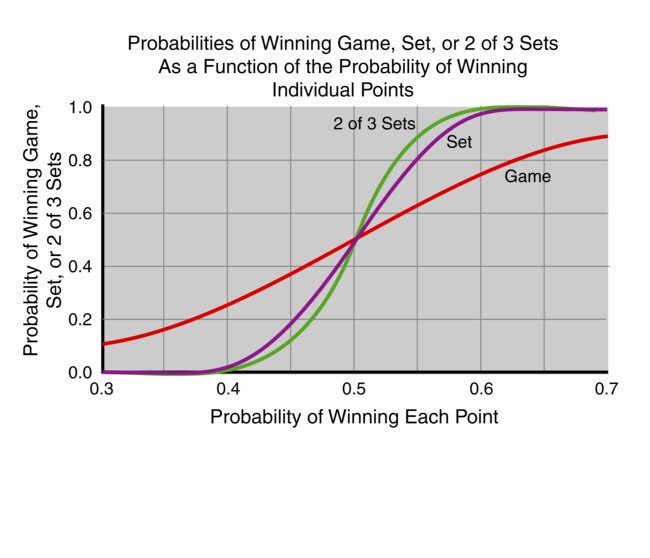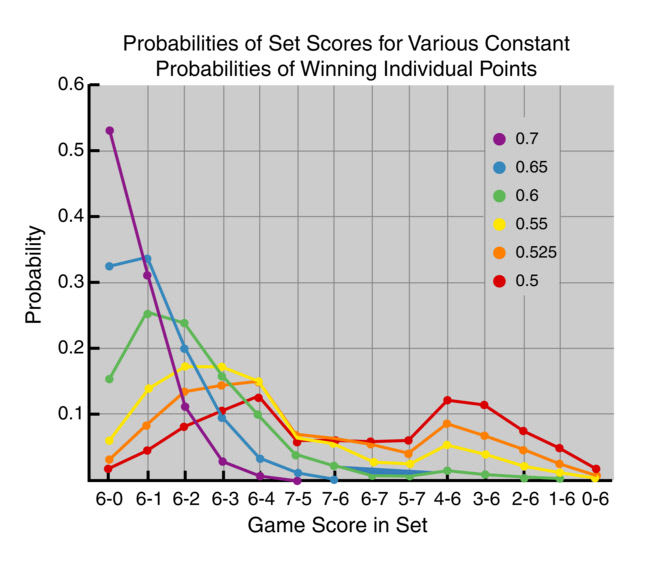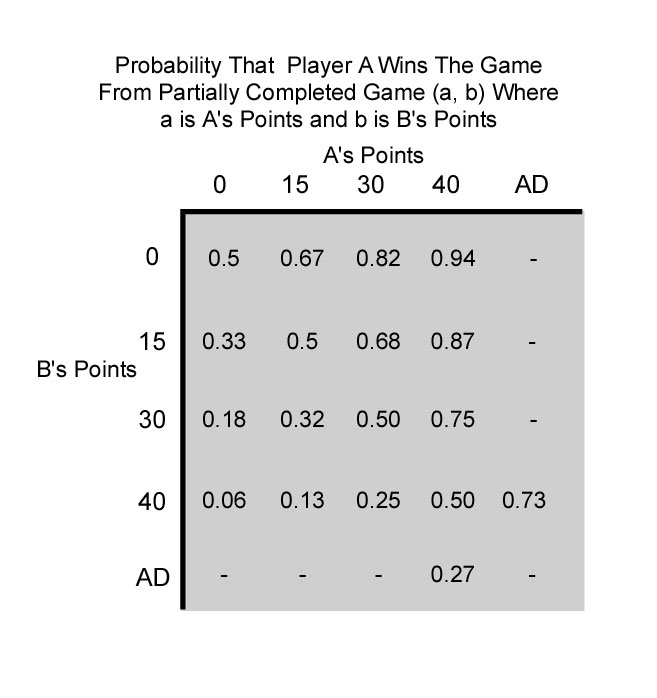Tennis Outcomes Related to Probabilities of Winning Individual Points

Suppose in every point of a match you had a 50% probability of winning every point. If you played thousands of such matches you would expect to win as many games, sets, and matches as you lose. Furthermore, you expect that much more of the time the scores will be close, like 6-4 rather than 6-0. What are the scores like and how often do you win for other probabilities of winning each point? The following graphs show the results for relevant probabilities. For example, if your probability of winning each point were 70%, your probability of winning a game is 91%, you virtually never lose a set, and 60% of the time you win a set at 6-0.These results were generated by the Python computer program shown below that used a random number generator to "play" points according to the different probabilities and which then kept score, using a 7 point tie-breaker at 6-6. For each probability, 10,000 matches were played. An interesting result is that even with a very modest improvement in one's probability of winning a point, one's probability of winning the match is very dramatically improved. For example, if one's probability of winning a point improves from 50% to 55%, the probability of winning a set improves from 50% to 84%.

As we get to smaller units, the statistical averages become less helpful in making strategic decisions. Nonetheless, at the game level, if one's probability of winning a point is 0.5, 0.6, 0.7, and 0.8, one's probability of winning a game is 0.5, 0.74, 0.91, and 0.98. It is also of interest to know in a partially completed game, what the probability is of winning the game. The following table shows the probability that A wins the game starting from the indicated points for player A and B. For example, from the score 30-40, A has a 25% chance of winning the game. Note that from a score of 40-Love, between equal players, A should win the game 95% of the time. In actual play, it seems like A actually wins the game less than 90% of the time. This discrepancy indicates that either or both A and B do not maintain the same level of intensity and concentration independent of the score.``````
#If file name of the script is tennis.py, run by typing "python tennis.py" at prompt.
#Python program that simulates 10,000 sets of tennis in which there is a uniform
#probability x where 0 < x < 1 of A winning each point. The program plays
#individual points having player A randomly win or lose a point depending on x.
#Games are played using deuce if necessary and sets will use a 7-point
#tiebreaker if 6-6 in games is reached. Note that the scoring structure of normal
#games and a tiebreaker game are identical except for the lowest winning score.
#Program counts scores as 0, 1, 2, 3 rather than the conventional 15, 30, 40.
#a and b are the points for players A and B, and A and B are their game scores.
#Typically invoke the program by typing "python tennis.py" at the prompt.
#Set scores go to screen and a file. Count number of specific scores, i.e. 6-3 (Linux)
#using "grep -oc '6 3' scores.txt"

import sys
import math
import random

prob = input("Enter probability x, 0 < x < 1 of A winning points, e.g. 0.525 ")
f = open('scores.txt', 'w')
for i in range(0, 10000):
#Adjust range limits for the desired number of sets played
game, A, B, = 3, 0, 0
#A set begins with the usual scoring for a game and zero games for the players
while True:
a, b = 0, 0
#Start game with zero points for each player
while True:
if(random.random() < prob):
a = a + 1
else:
b = b + 1
if(a > game and a - b >= 2):
A = A + 1
break
if(b > game and b - a >= 2):
B = B + 1
break
#This logic handles games that do or do not reach deuce as well as tiebreakers
#           print a, b, A, B
if((A > 5 and A - B >= 2) or (B > 5 and B-A >= 2)):
#A set is won by "First to six, leading by at least 2"
break
if((A == 7 and B == 6) or (B == 7 and A == 6)):
break
if(A == 6 and B == 6):
game = 6
#At 6 games all, a tiebreak game is to be played, and minimum score to
#win the "tiebreak game" becomes 7.
else:
continue
print A, B
f.write(str(A) + ' ' + str(B) + "\n" )
print "Output will also be in the file 'scores.txt'"
f.close()

``````
``` ```